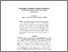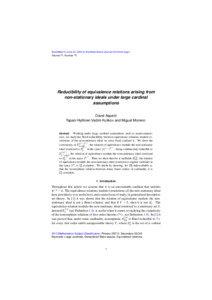# Reducibility of equivalence relations arising from non-stationary ideals under large cardinal assumptions

Aspero, David, Hyttinen, Tapani, Kulikov, Vadim and Moreno, Miguel (2019) Reducibility of equivalence relations arising from non-stationary ideals under large cardinal assumptions. Notre Dame Journal for Formal Logic, 60 (4). pp. 665-682.Preview PDF (AHKM_June) - Accepted Version Download (389kB) | Preview

## Abstract

Working under large cardinal assumptions, we study the Borel-reducibility between equivalence relations modulo restrictions of the non-stationary ideal on some fixed cardinal \kappa. We show the consistency of E^{\lambda^{++},\lambda^{++}}_{\lambda\text{-club}}, the relation of equivalence modulo the non-stationary ideal restricted to S^{\lambda^{++}}_\lambda in the space (\lambda^{++})^{\lambda^{++}}, being continuously reducible to E^{2,\lambda^{++}}_{\lambda^+\text{-club}}, the relation of equivalence modulo the non-stationary ideal restricted to S^{\lambda^{++}}_{\lambda^+} in the space 2^{\lambda^{++}}. Then we show that for \kappa ineffable E^{2, \kappa}_{\text{reg}}, the relation of equivalence modulo the non-stationary ideal restricted to regular cardinals in the space 2^{\kappa}, is \Sigma^1_1-complete. We finish by showing, for \Pi_2^1-indescribable \kappa, that the isomorphism relation between dense linear orders of cardinality \kappa is \Sigma^1_1-complete.

Item Type: Article Faculty of Science > School of Mathematics Faculty of Science > Research Groups > Logic LivePure Connector 16 Jan 2019 09:30 21 Oct 2022 21:34 https://ueaeprints.uea.ac.uk/id/eprint/69586 10.1215/00294527-2019-0024View Item